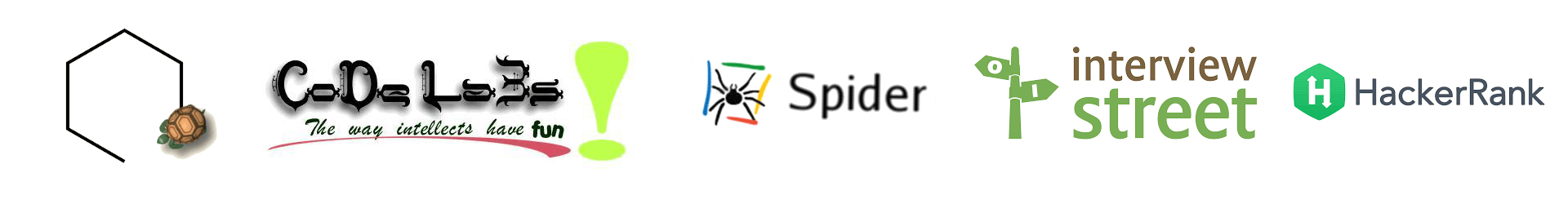I see, learn and rediscover… everyday!I don’t remember who exactly gave me this script. Must be either Verma or Manas, but this one is so cool.
The code looks pretty easy and clear, though I didn’t try to understand it completely.
So this is what you have to do.

Open Google image search and search for some image. If you are lazy to do that, click

Then copy this javascript code and paste it in the URL bar
`javascript:R=0; x1=.1; y1=.05; x2=.25; y2=.24; x3=1.6; y3=.24; x4=300; y4=200; x5=300; y5=200; DI= document.images; DIL=DI.length; function A(){for(i=0; i<DIL; i++){DIS=DI[ i ].style; DIS.position='absolute'; DIS.left=Math.sin(R*x1+i*x2+x3)*x4+x5; DIS.top=Math.cos(R*y1+i*y2+y3)*y4+y5}R++}setInterval('A()',5); void(0)`

Cool right? 😉

1.Alagappan

That was really cool…

Is there a way to stop it without closing the window??

2.sp2hari

Good question 🙂

This script stops the images at current position.

javascript:function A(){}

And obviously, the following script will stop the movement and put the images in the respective position 😉